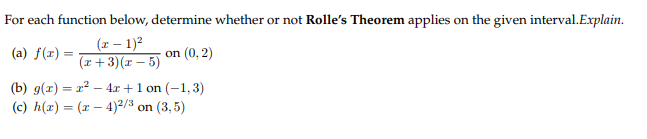# For each function below, determine whether or not Rolle's Theorem applies on the given interval.Explain.(-12(Eon (0.3)( 1 3r 5)(U)(b) g(x)2 41 on (-1,3)(c) h(z) ( 4)2/ on (3,5)

Question
1 viewshelp_outlineImage TranscriptioncloseFor each function below, determine whether or not Rolle's Theorem applies on the given interval.Explain. (-12 (Eon (0.3) ( 1 3r 5)(U) (b) g(x)2 41 on (-1,3) (c) h(z) ( 4)2/ on (3,5) fullscreen
check_circle

Step 1

To validate or otherwise Rolle's theorem for the three given functions

Step 2

Recall the statement of Rolle's Theorem: If f(x) is differentiable on (a,b) and f(a) =f(b), then there exists an interior point c , where the tangent is parallel to the x-axis (f'(c)=0)

Step 3

*a) Appicable ...

### Want to see the full answer?

See Solution

#### Want to see this answer and more?

Solutions are written by subject experts who are available 24/7. Questions are typically answered within 1 hour.*

See Solution
*Response times may vary by subject and question.
Tagged in

### Calculus